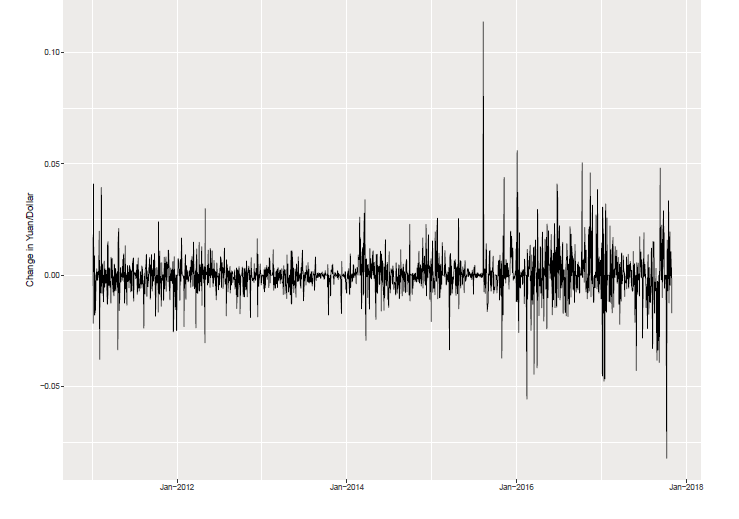Economics Tutors | Micro, Macro & Econometrics Tuitions | EconTutors

# PhD Econometrics Tutors in New York

Work with our PhD Econometrics Tutors in New York to prepare for basic undergrad econometrics, or advanced topics in econometrics. Our tutors tailor each session according to your gaps in understanding. A lack of sound statistical understanding is the most frequently cited roadblock for understanding econometrics. We have a statistical package prepared for students to focus on the foundational concepts before they can begin working on econometrics.1. Becoming acquainted with the assumptions of the simple linear regression model.

2. Understanding how an estimator uses sample data to calculate values of interest.

3. Learning how consistency, unbiasedness and efficiency are sought-after characteristics of estimators.

4. Evaluating various error functions on their ability to estimate a regression line.

5. Deriving explicit formulas for the slope and intercept parameters in ordinary least squares (OLS) regression.

6. Deriving explicit formulas for the variance of the slope and intercept parameters in ordinary least squares (OLS) regression.

#### Statistical review

1. Understanding how to calculate confidence intervals and conduct hypothesis tests on the regression coefficients B1 and B2.
2. Understanding how to calculate confidence intervals and conduct hypothesis tests on the expected value of Y, for a given X-value.
3. Becoming acquainted with indicator variables (or dummy variables) in a regression.
4. Learning how indicator variables can be used in a simple linear regression to run two-population hypothesis tests.
5. Becoming acquainted with prediction intervals and how they differ from confidence intervals.
6. Learning how to compute an R2-value and understanding its relation to correlation coefficients.
7. Gaining familiarity with some nonlinear functional forms.

#### The null vs alternative hypothesis

The data suggest 1Q kids get less schooling, but the observed difference is just 0.08 years so maybe it’s just sampling variation.

Is the observed difference real or is it due to chance?

The null hypothesis (H0) says that an observed difference reflects pure chance variation.

The alternative hypothesis (H1 or Ha) says that the observed difference is real.

Econometriccs Stats Review

Specialist-Econometrics-Tutors-UK

AP-Microeconomics-Tutors-newyork

macroeconomics-tutor-new-york-nyu-yale-columbia-princeton

macroeconomics-tutor-nyu-columbia-yale-princeton

finance-tutor-new-york

mba-microeconomics-tutor-newyork

statistics-tutor-newyork

economics-tutor-dubai-sharjah-uae-aus-online

labor-economics-urban-economics-public-economics-tutor-newyork-nyu-columbia-nyc

economics-tutor-degree-level

Microeconomics Tutors NYU

Macroeconomics Tutors NYU

Econometrics Tutors NYU

Finance Tutors NYU

Reviews

Get Started

## See the #1 economics mentoring platform in action

Request a demo